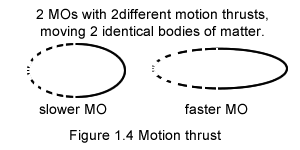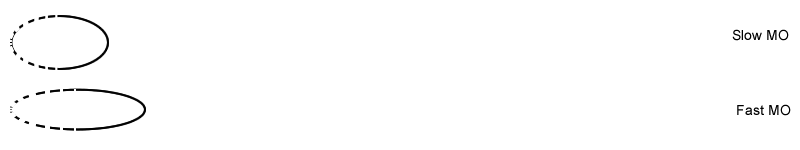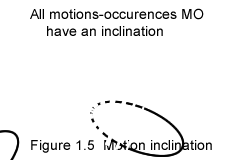Motion-thrust and the equalization phenomenon (§1.4)Motion-thrust (§1.4.1)
The intensity of a given motion is called motion-thrust in gravimotion.
The motion thrust is represented through the elongation of the MO.
Note the motion thrust doesn't fit our concept of speed; speed is expressed in terms of miles (or kilometers) per hour, and neither distance (miles) nor time (hours) is participating to the motion that occurs behind the word speed.
Motion is so natural and evident that our human mind doesn't even notice the discrepancy there is between the reality of motion and our mental concept of speed.
A motion-occurrence is a live entity, constantly out of equilibrium, constantly tumbling end over end, constantly seeking equilibrium.

Besides uniform motion, as shown later in the section (§4.4 Model of free fall or gravimotion), a motion occurence may also represent an acceleration.
In gravimotion an acceleration is motion.
Equalization phenomenon (versus entropy §1.4.2)
When a larger system is out of equilibrium, it is also seeking equilibrium; the phenomenon is given the name of equalization phenomenon in gravimotion.
A motion occurrence is a small scale equalization phenomenon.
Gravimotion's
equalization phenomenon replaces physics' entropy
Gravimotion's equalization phenomenon versus physics' entropy
At the origin of physics' entropy is the allegation that heat is always moving from hot to cold, and that heat never reverts from cold to hot. But that is biased analysis. The fact is that in a transfer of heat as many molecules are heated on the cold side (as much heat goes toward the cold) as molecules are cooled on the hot side (as coldness goes toward the hot side).
So equalization (of motions of molecules) rather than degradation (of energy) is taking place.
Besides energy degradation as conceived in the "entropy concept", is in direct conflict with physics' very principle of conservation of energy, as degradation implies "reduction" in strength or intensity.
Gravimotion's equalization of motions is much more realistic.
.
Entropy is mandated in physics as it justifies the arrow of time. The entropy law (2nd thermodynamic law) compels things to occur along time, which the conservation of energy law (1st thermodynamic law) doesn't provide.
Yet there is no need to integrate into "degradation of energy" the motion of a great number of molecules to justify time. In gravimotion should time exist it would be a byproduct of the motion occurrence.
From gravimotion's point of view though, entropy major deficiency is that it has been designed forgetting all about gravity! And ignoring such hindrance, physicists nevertheless assert that, due to entropy, the universe will end in a dull thermal equilibrium; yet universal gravity at work will no doubt modify such prediction!
As a matter of fact is it not time to blow the whistle?
Physicists appear surprised that gravity is not part of the quantum theory!

As the following shows, the lack of gravity in the quantum theory is intrinsic to the theory very foundation. Planck the father of the theory explained black body's radiation, through Boltzmann entropy equation to which he added an invention of his that of the quantum. Yet Boltzmann equation was based on both Maxwell invention of statistics and Rudolph Clausius concept of entropy; and Clausius based his concept of entropy on Sadi Carnot declaration that a transfer of heat is a degradation of energy.

Where in all of that is gravity?

The reason for which gravity is foreign to the quantum theory is plain and simple. Gravity has been excluded from quantum theory inception to this day! Even the Higgs boson introduced in the 1960's seems to be mathematically designed independently of gravity.

Einstein main argument against quantum theory was to say that it is incomplete. He was referring to the fact that the behavior of the electrons was missing in quantum mechanics entanglement equations; while the equations provide a statistical behavior of the electrons, these very electrons are absent from these equations; the detectors settings only determine the outcome.
Einstein might have added that the quantum theory has been designed forgetting all about gravity.
Motion-inclination (§1.5)In science the direction of any motion is defined with respect to a reference system independent of the motion observed. As an example, we walk in reference to the ground, which can be used to define a reference system.
Yet we also are walking straight ahead or sideways, now with respect to our own vision and eyes.
A motion-occurrence doesn't have a direction in space but an internal inclination.
The 2 motion-occurrences of this new figure could represent 2 human beings each moving straight ahead (having the same inclination) yet walking into 2 different directions as defined in physics.
Gravimotion's definition of inclination parallels somewhat physics' irreversibility (unique direction) of time.
Because the inclination is internal to a motion occurrence, motion inclination and thrust make one; an MO internal inclination is also shown through the elongation of the MO.
As explained in a later section (§1.8 Cyclic motions) the inclination of a MO is not restricted as being straight, it might also be curved.

Even though it has no equivalent in physics, this concept of internal inclination is primordial.
Here is why. Uniform motion in Newton's first law, is justified by the inertia (or inertial-mass) of the body in motion. Yet neither our concept of inertia nor that of mass has direction. How then does science explain that a body in uniform motion keeps its direction unchanged?
Worse, an object spinning under the influence of inertia, because constantly changing direction, contradicts that law!
Besides stating its occurrence, the science of physics eludes the uniform motion fundamental phenomenon; its ramifications end up split in a number of incompatible mathematics'.

>